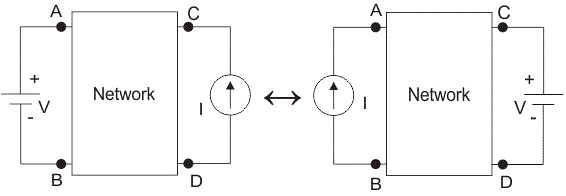# Reciprocity Theorem

## What is Reciprocal Property?

In many electrical networks it is found that if the positions of voltage source and ammeter are interchanged, the reading of ammeter remains the same. It is not clear to you. Let’s explain it in details. Suppose a voltage source is connected to a passive network and an ammeter is connected to other part of the network to indicate the response.
Now any one interchanges the positions of ammeter and voltage source that means he or she connects the voltage source at the part of the network where the ammeter was connected and connects ammeter to that part of the network where the voltage source was connected.

The response of the ammeter means current through the ammeter would be the same in both the cases. This is where the property of reciprocity comes in the circuit. The particular circuit that has this reciprocal property, is called reciprocal circuit. This type of circuit perfectly obeys reciprocity theorem.

## Explanation of Reciprocity Theorem

The voltage source and the ammeter used in this theorem must be ideal. That means the internal resistance of both the voltage source and ammetermust be zero. The reciprocal circuit may be a simple or complex network. But every complex reciprocal passive network can be simplified into a simple network. As per reciprocity theorem, in a linear passive network, supply voltage V and output current I are mutually transferable.

The ratio of V and I is called the transfer resistance. The theorem can easily be understood by this following example.Want To Learn Faster? 🎓
Get electrical articles delivered to your inbox every week.
No credit card required—it’s 100% free.